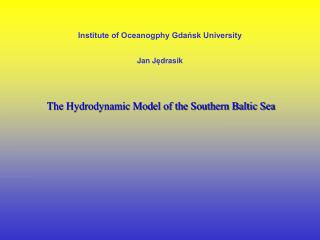DownloadDownload PresentationInstitute of Oceanogphy Gdańsk University J an J ę drasik

# Institute of Oceanogphy Gdańsk University J an J ę drasik

Télécharger la présentation## Institute of Oceanogphy Gdańsk University J an J ę drasik

- - - - - - - - - - - - - - - - - - - - - - - - - - - E N D - - - - - - - - - - - - - - - - - - - - - - - - - - -
##### Presentation Transcript

1. Institute of Oceanogphy Gdańsk University Jan Jędrasik The Hydrodynamic Model of the Southern Baltic Sea

2. The hydrodynamic model • Based on Princeton Ocean Model (Blumberg and Mellor 1987) • Vertical mixing processes are parameterized by the scheme of second order turbulence closure (Mellor and Yamada 1982) • In order to apply the model for the Baltic Sea some modifications were done (Kowalewski 1997)

3. Descriptionof the hydrodynamic modelM3D_UG Equations and boundary cionditions where: u, v, w,componentsof velocity prędkości;f, Coriolis parameter; , 0, density of sea water in situand reference density; g, gravity acceleration; p, pressure; KM, AM, vertical and horizontal viscosity coefficients where: patm, atmospheric preassure; , sea level elevations where: T, temperatureof water; S, salinity; KH, AH,vertical and horizontal diffusivity coefficients; T, sourcesof heat where: AC,,empirical coefficient; x, y, spatial steps in xand y direction. where: q2, turbulent kinetic energy, turbulent macroscale; Kq, coefficient of vertical exchange of turbulent energy; , Karman‘s constant; H, sea depth; B1, E1, E2, empirical constants.

4. At the sea surface Heat fluxes Energy fluxes Kinematic condition at the surface At the bottom z = H Parametrised as Fluxes of energy at the bottom Kinematic condition at the bottom where: ox, oy, wind surface stresses; H0, heat fluxes from atmosphere; bx, by, bottom stresses; CD, drag coefficient (CD=0.0025); friction velocity; u, ub, v, vb, w, wb, components of velocity at the surface (no index) and at the bottom (with b index). At the lateral boundary (rivers) u(x,y,z) = 0, v(x,y,z) = 0, w(x,y,z) = 0 Initial conditions T = T(x,y,z), S = S(x,y,z).

5. Application of the model Rotational criterium where: , angular velocityof Earth; , geographical latitude Horizontal diffusivity criterium where: AH, horizontal diffusivity coefficient Courant-Fridrichs-Levy’s condition where: C velocity of fundamental mode, Umax , maxime current velocity; or Ct = 2Ci + umax, Ci , velocity of fundamental internal mode, umax , maxime advection velocity. Radiation condition Sigma coordinates (x*, y*, , t*), x* = x,    y* = y,    ,   t* = t, where: D = H + , dla z =  = 0, for z = -H  = -1

6. The modelled areas • The inflows from 85 rivers • The fields of wind speed over the sea surface were taken from 48-hours ICM forecast model

7. Area I Area II Area III Numerical grids Vertical grid • based on -transformation defined as: Horizontal grid • Model allows to define subareas with different grid density

8. Temporal and spatial steps in the modelled areas